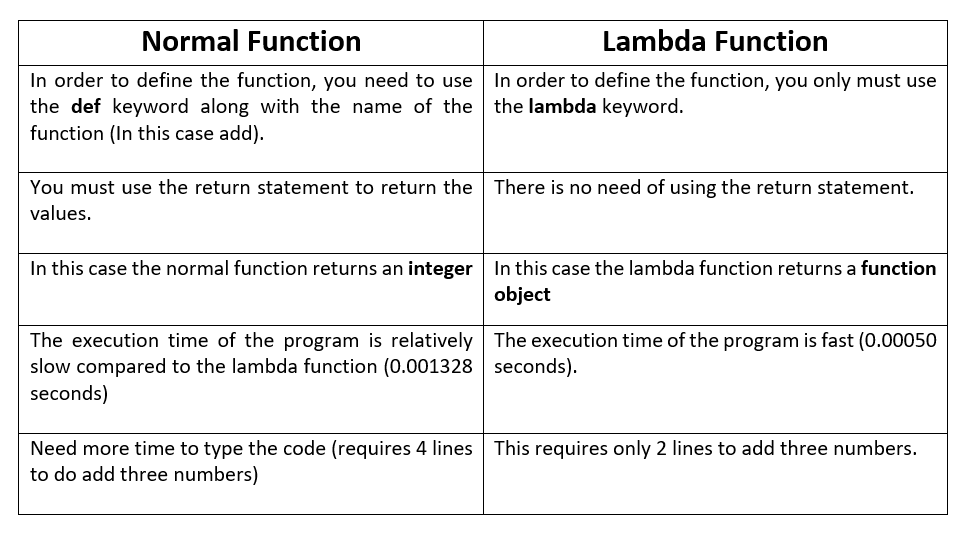# LAMBDA FUNCTION IN PYTHONLambda function in python is an anonymous function that takes any number of arguments but takes only one expression. An anonymous function is a function defined without a name.

``````SYNTAX
lambda arguments: expression``````

Normal functions are defined using the def keyword whereas anonymous functions are defined using the lambda keyword.

##### EXAMPLE 01: To add two numbers
``````>>> x = lambda a, b : a + b
>>> print(x(3,4))

Output:
7``````
##### EXAMPLE 02: To multiply three numbers
``````>>> x = lambda a, b : a * b * c
>>> print(x(3,4,2))

Output:
24``````##### WHY LAMBDA FUNCTION ??

Lambda function in python is used when we need a nameless function for a short period of time. We generally use lambda function inside another function. Let’s take an example:

``````>>> def normal_function(n):
return lambda a : a + n
>>> callfn = normal_function(2)
>>> print(callfn(5))

Output: 7``````

Lambda functions can be used together with Python’s built-in functions like map( ), filter( ), reduce( ), etc.

##### LAMBDA WITH FILTER( )

The filter() function takes a lambda function together with a list as the arguments. This function can be used to filter out all the elements of the list which satisfies the condition and returns a new list.

``````# filter() with lambda()
# create list of number divisible by 3
>>> list_01 = [1,2,3,4,5,6,7,8,9,10]
>>> new_list = list(filter(lambda x: (x%3 == 0) , list_01))
>>> print(new_list)

Output:
[3,6,9]``````
##### LAMBDA WITH MAP( )

The map() function takes a function together with a list as the argument. This function can be used to generate elements of a list returned by that function for each item.

``````>>> original_list = [2,4,6,8,10,12,14,16]
>>> new_list = list(map(lambda x: x * 2 , original_list))
>>> print(new_list)

Output:
[1,3,5,7,9,11,13,15]``````
##### LAMBDA WITH REDUCE( )

The reduce() function takes a function together with a list as the argument. We obtain a reduced output using this function. This is a part of functools module. Let’s take a look at an example:

``````>>> from functools import reduce
>>> old = [1,2,3,4,5,6,7,8,9,10]
>>> sum = reduce((lambda x, y: x + y), old)
>>> print (‘Sum of the first ten natural number is :’,sum)

Output:
Sum of the first ten natural number is 55``````

Process involved = (((((((((1+2)+3)+4)+5)+6)+7)+8)+9)+10) = 55. Follow us on instagram for more contents.

This page is contributed by Diwas & Sunil . If you like AIHUB and would like to contribute, you can also write an article & mail your article to  itsaihub@gmail.com . See your articles appearing on AI HUB platform and help other AI Enthusiast.🚀 I'm Diwas Pandey, a Computer Engineer with an unyielding passion for Artificial Intelligence, currently pursuing a Master's in Computer Science at Washington State University, USA. As a dedicated blogger at AIHUBPROJECTS.COM, I share insights into the cutting-edge developments in AI, and as a Freelancer, I leverage my technical expertise to craft innovative solutions. Join me in bridging the gap between technology and healthcare as we shape a brighter future together! 🌍🤖🔬

View all posts by Diwas →

### 5 Comments on “LAMBDA FUNCTION IN PYTHON”

1.adreamoftrains best hosting says:

Very nice write-up. I definitely love this site. Stick with it!
2.web hosting providers says:
3.best web hosting says: